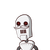# d. Write the following in exponential form.( 2/5) ^-2 ×( 2/5) ^-2​

d. Write the following in exponential form.
( 2/5) ^-2 ×( 2/5) ^-2​

### 1 thought on “d. Write the following in exponential form.<br />( 2/5) ^-2 ×( 2/5) ^-2​”

1.$${( \frac{2}{5}) }^{ – 4} \\$$

Step-by-step explanation:

$$( \frac{2}{5} ) ^{ – 2} \times {( \frac{2}{5} )}^{ – 2} \\ = {( \frac{2}{5} )}^{ – 2 + ( – 2)} \\ = {( \frac{2}{5} )}^{ – 2 – 2} \\ = {( \frac{2}{5} )}^{ – 4}$$

it done by the formula :

$${x}^{m} \times {x}^{n} = {x}^{m + n}$$

Where bases are same and we have to multiply them, we will add the powers.

more formulas to remember :

$${x}^{m} \div {x}^{n} = {x}^{m – n} \\ ( {x}^{m} )^{n} = {x}^{m \times n}$$

hope it helps.